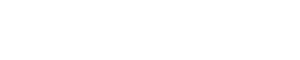Drag

# 数学代写｜MATH569 Statistical Learning Final Exam (Take Home)

## Instructions:

i.This exam consists of FIVE problems. Answer all of them.

ii.This exam is take-home exam. You can use any books or notes to help you answer the questions. But you MUST fifinish the exam INDEPENDENTLY, without discussing with anybody else.

iiii.There are TWO data analysis problems. You should reformat the computer outputs when answering the questions. Do not directly paste the computer outputs.

Mathematical problems

Problem 1. (20 points 10-10)

Let X1 R and X2 R be random variables and

Y = m(X1, X2) + 

where E( ) = 0 and E( 2 ) = σ2.

(a) Consider the class of multiplicative predictors of the form m(x1, x2) = βx1x2. Let βbe the best predictor, that is, βminimizes EY,X1,X2 (Y βX1X2)2 . Find an expression for β.

(b) Suppose the true regression function is

Y = X1 + X2 + .

Also assume that E(X1) = E(X2) = 0, E(X12 ) = E(X22 ) = 1 and that X1 and X2 are independent.

Find the predictive risk R = E(Y βX1X2)2 where βwas defifined in (a).

Problem 2. (10 points)

Show that for the linear regression model Y = XT β +  , the leave-one-out cross validation identity:where H = X(XT X)1XT is the hat matrix and Hii is the ith diagonal entry, Yˆi is the ith prediction value for the training point xi and Yˆ(i) is the leave-one-out prediction at xi.

Problem 3. (20 points 5-5-5-5)

Let (Z1, Y1), . . . , (Zn, Yn) be generated as follows:

Zi Bernoulli(p)

Yi  N(0, 1)   ifZi = 0

N(5, 1)    ifZi = 1

(a) Assume we do not observe the Zi ’s. Write the pdf f(y) of Y as a mixture of two normal distribution pdf. (Use the notation φ(·) which is the standard normal pdf.)

(b) Write down the likelihood function for p (without Zi ’s).

(c) Write down the complete likelihood function for p (assuming the Zi ’s are observed).

(d) Find the maximum likelihood estimation of p using the likelihood from (c).